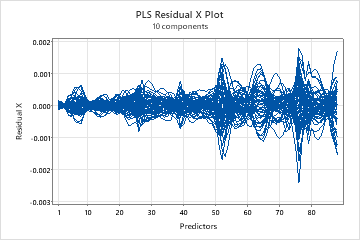# Interpret the key results for Partial Least Squares Regression

## Step 1. Determine the number of components in the model

The objective with PLS is to select a model with the appropriate number of components that has good predictive ability. When you fit a PLS model, you can perform cross-validation to help you determine the optimal number of components in the model. With cross-validation, Minitab selects the model with the highest predicted R2 value. If you do not use cross-validation, you can specify the number of components to include in the model or use the default number of components. The default number of components is 10 or the number of predictors in your data, whichever is less. Examine the Method table to determine how many components Minitab included in the model. You can also examine the Model selection plot.

When using PLS, select a model with the smallest number of components that explain a sufficient amount of variability in the predictors and the responses. To determine the number of components that is best for your data, examine the Model selection table, including the X-variance, R2, and predicted R2 values. Predicted R2 indicates the predictive ability of the model and is only displayed if you perform cross-validation.

In some cases, you may decide to use a different model than the one initially selected by Minitab. If you used cross-validation, compare the R2 and predicted R2. Consider an example where removing two components from the model that Minitab only slightly decreases predicted R2. Because the predicted R2 only decreased slightly, the model is not overfit and you may decide it better suits your data.

A predicted R2 that is substantially less than R2 may indicate that the model is over-fit. An over-fit model occurs when you add terms or components for effects that are not important in the population, although they may appear important in the sample data. The model becomes tailored to the sample data and, therefore, may not be useful for making predictions about the population.

If you do not use cross-validation, you can examine the x-variance values in the Model selection table to determine how much variance in the response is explained by each model.

## Step 2. Determine whether the data contain outliers or leverage points

To determine whether your model fits the data well, you need to examine plots to look for outliers, leverage points, and other patterns. If your data contain many outliers or leverage points, the model may not make valid predictions.

You can examine the residual plots, including the residuals vs leverage plot. On the residuals vs leverage plot, look for the following:
• Outliers: Observations with large standardized residuals fall outside the horizontal reference lines on the plot.
• Leverage points: Observations with leverage values have x-scores far from zero and are to the right of the vertical reference line.

For more information on the residual vs leverage plot, go to Graphs for Partial Least Squares Regression.

In this plot, there are two points that may be leverage points because they are to the right of the vertical line. There are three points that may be outliers because they are above and below the horizontal reference lines. These points can be investigated to determine how they affect the model fit.You can also examine the Response plot to determine how well the model fits and predicts each observation. When examining this plot, look for the following things:
• A nonlinear pattern in the points, which indicates the model may not fit or predict data well.
• If you perform cross-validation, large differences in the fitted and the cross-validated values, which indicate a leverage point.
In this plot, the points generally follow a linear pattern, indicating that the model fits the data well. The points that appear on the residual vs leverage plot above do not seem to be an issue on this plot.In this plot, cross-validation was used so both the fitted and cross-validated fitted values appear on the plot. The plot does not reveal large differences between the fitted and cross-validated fitted responses.## Step 3. Validate the PLS model with a test data set

Often, PLS regression is performed in two steps. The first step, sometimes called training, involves calculating a PLS regression model for a sample data set (also called a training data set). The second step involves validating this model with a different set of data, often called a test data set. To validate the model with the test data set, enter the columns of the test data in the Prediction sub-dialog box. Minitab calculates new response values for each observation in the test data set and compares the predicted response to the actual response. Based on the comparison, Minitab calculates the test R2, which indicates the model's ability to predict new responses. Higher test R 2 values indicate the model has greater predictive ability.

If you use cross-validation, compare the test R2 to the predicted R2. Ideally, these values should be similar. A test R2 that is significantly smaller than the predicted R2 indicates that cross-validation is overly optimistic about the model's predictive ability or that the two data samples are from different populations.

If the test data set does not include response values, then Minitab does not calculate a test R2.

By using this site you agree to the use of cookies for analytics and personalized content.  Read our policy